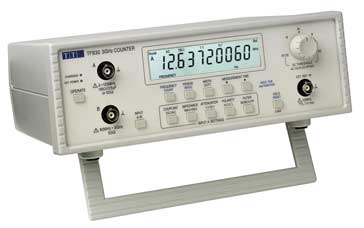# Problem Solving Patterns

When talking about the patterns, there will not the best problem-solving patterns it does not exist. Instead, look at all the patterns and choose whichever is best.

## 1. Frequency Counter​This will not calculate the frequency. This will collect the frequencies of value. It will use Objects/Sets of key and values to store the values. This can often avoid the need for nested loops or O(N^2) operations with arrays/strings.

Let's take an example:

• Write a function called sameValues, which accepts two arrays. The function should return true if every value in the array has its corresponding value squared in the second array. The frequency of values must be the same.

Sample input/outputs:

sameValues([1,2,3], [4,1,9]) // truesameValues([1,2,3], [1,9]) // falsesameValues([1,2,1], [4,4,1]) // false (must be same frequency)

Here is the problem the squarred value not only should be in second array. Instead it should be in same frequency/occurence

### Standard Solution:​

function same(arr1, arr2){    if(arr1.length !== arr2.length){        return false;    }    for(let i = 0; i < arr1.length; i++){        let correctIndex = arr2.indexOf(arr1[i] ** 2) // Loop inside a loop        if(correctIndex === -1) {            return false;        }        arr2.splice(correctIndex,1)    }    return true}// Time Complexity => O(N^2)

### Optimized solution​

function same(arr1, arr2){    if(arr1.length !== arr2.length){ // If array 1 is not equal to array 2 return false        return false;    }    // Initialize the freqeuncyCounter for both values    let frequencyCounter1 = {}    let frequencyCounter2 = {}        // Get array elements one by one and add 1 to it if already exists or else initiate it.     for(let val of arr1){        frequencyCounter1[val] = (frequencyCounter1[val] || 0) + 1    }    // Same applicable to array 2    for(let val of arr2){        frequencyCounter2[val] = (frequencyCounter2[val] || 0) + 1            }    // Now the important part, we checked each key in frequencyCounter1    // Squaring the value and check if it exists in frequencyCounter2    // If not return false    // And also check the passed key having the same frequency if not return false.    // If it pass through all the conditions return true.    for(let key in frequencyCounter1){        if(!(key ** 2 in frequencyCounter2)){            return false        }        if(frequencyCounter2[key ** 2] !== frequencyCounter1[key]){            return false        }    }    return true}// Time Complexity => O(n)

In this problem, we removed the loop inside the loop instead we created the separate loops and store the values in the object.

## 2. Multiple Pointers​

Creating pointers or values that correspond to an index or position and move towards the beginning, end or middle based on a certain condition

Very efficient for solving problems with minimal space complexity as well

#### Example:​

Write a function called sumZero which accepts a sorted array of integers. The function should find the first pair where the sum is 0. Return an array that includes both values that sum to zero or undefined if a pair does not exist

Sample Inputs/Outputs

sumZero([-3,-2,-1,0,1,2,3]) // [-3,3] sumZero([-2,0,1,3]) // undefinedsumZero([1,2,3]) // undefined

### Standard Solution:​

function sumZero(arr){    for(let i = 0; i < arr.length; i++){        for(let j = i+1; j < arr.length; j++){ // Loop inside a loop            if(arr[i] + arr[j] === 0){                return [arr[i], arr[j]];            }        }    }}// Time Complexity => O(n^2)

### Optimized Solution:​

function sumZero(arr){    let left = 0; // Initialize the first pointer with 0th index    let right = arr.length - 1; // Second pointer to the end of the array    while(left < right){ // Iterate till the two pointer become same        let sum = arr[left] + arr[right];         if(sum === 0){ // If sum zero found the values return it.            return [arr[left], arr[right]];        } else if(sum > 0){ // Means the right pointer value will not match anymore so we need to decrease our array order.             right--;        } else { // the left pointer value not be found in right pointer value            left++;        }    }}

## 3. Sliding Window​

• This pattern involves creating a window which can either be an array or number from one position to another

• All the way from beginning to end it's sliding one another. First, it'll solve the first set of values and then go to the next set. Will continue till the end.

• Depending on a certain condition, the window either increases or closes (and a new window is created)

• Very useful for keeping track of a subset of data in an array/string etc.

#### Example:​

Write a function called maxSubarraySum which accepts an array of integers and a number called n. The function should calculate the maximum sum of n consecutive elements in the array.

Sample Inputs: maxSubarraySum([1,2,5,2,8,1,5],2) // 10 maxSubarraySum([1,2,5,2,8,1,5],4) // 17 maxSubarraySum([4,2,1,6],1) // 6 maxSubarraySum([4,2,1,6,2],4) // 13 maxSubarraySum([],4) // null

### Standard Solution​

function maxSubarraySum(arr, num) {  if ( num > arr.length){    return null;  }  var max = -Infinity;  for (let i = 0; i < arr.length - num + 1; i ++){    temp = 0;    for (let j = 0; j < num; j++){      temp += arr[i + j];    }    if (temp > max) {      max = temp;    }  }  return max;}

### Optimized solution​

function maxSubarraySum(arr, num){  let maxSum = 0;  let tempSum = 0;  if (arr.length < num) return null;  for (let i = 0; i < num; i++) { // Initiated out first slide with num numbers    maxSum += arr[i]; // Store the sum on num array values in maxSum  }  tempSum = maxSum; // Initiate the tempSum with maxSum value  for (let i = num; i < arr.length; i++) { // Iterate from where you left before    tempSum = tempSum - arr[i - num] + arr[i]; // don't need to iterate again the num numbers of next slide instead sub the earlier starting value and add next first value.    maxSum = Math.max(maxSum, tempSum); // Calculate the maximum of two values and asign to maxSum  }  return maxSum;}